# BS PPT.ppt

30 de Sep de 2022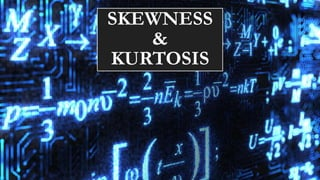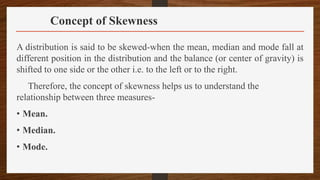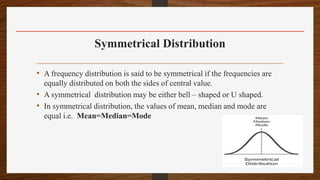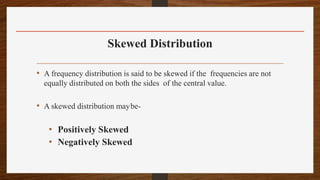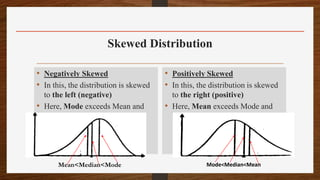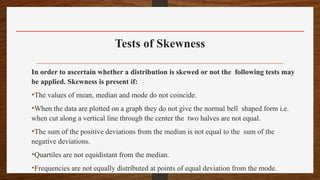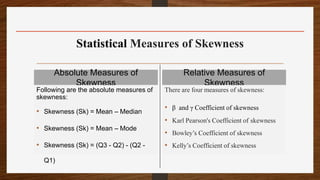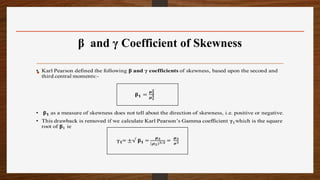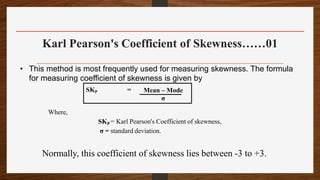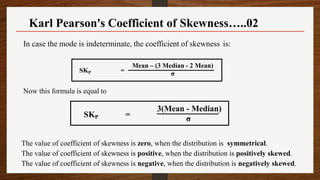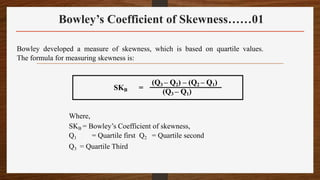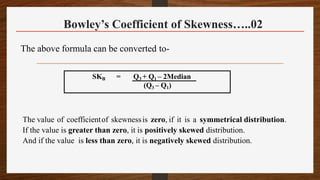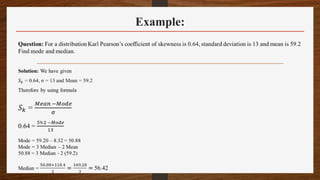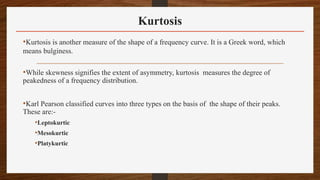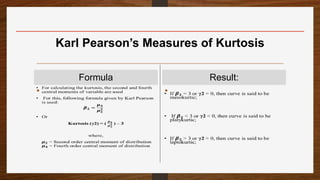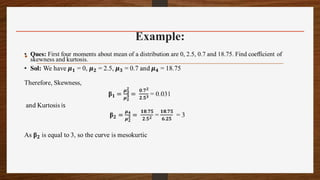1 de 17

### BS PPT.ppt

• 2. Concept of Skewness A distribution is said to be skewed-when the mean, median and mode fall at different position in the distribution and the balance (or center of gravity) is shifted to one side or the other i.e. to the left or to the right. Therefore, the concept of skewness helps us to understand the relationship between three measures- • Mean. • Median. • Mode.
• 3. Symmetrical Distribution • A frequency distribution is said to be symmetrical if the frequencies are equally distributed on both the sides of central value. • A symmetrical distribution may be either bell – shaped or U shaped. • In symmetrical distribution, the values of mean, median and mode are equal i.e. Mean=Median=Mode
• 4. Skewed Distribution • A frequency distribution is said to be skewed if the frequencies are not equally distributed on both the sides of the central value. • A skewed distribution maybe- • Positively Skewed • Negatively Skewed
• 5. Skewed Distribution • Negatively Skewed • In this, the distribution is skewed to the left (negative) • Here, Mode exceeds Mean and Median. • Positively Skewed • In this, the distribution is skewed to the right (positive) • Here, Mean exceeds Mode and Median. Mean<Median<Mode Mode<Median<Mean
• 6. Tests of Skewness In order to ascertain whether a distribution is skewed or not the following tests may be applied. Skewness is present if: •The values of mean, median and mode do not coincide. •When the data are plotted on a graph they do not give the normal bell shaped form i.e. when cut along a vertical line through the center the two halves are not equal. •The sum of the positive deviations from the median is not equal to the sum of the negative deviations. •Quartiles are not equidistant from the median. •Frequencies are not equally distributed at points of equal deviation from the mode.
• 7. Statistical Measures of Skewness Absolute Measures of Skewness Following are the absolute measures of skewness: • Skewness (Sk) = Mean – Median • Skewness (Sk) = Mean – Mode • Skewness (Sk) = (Q3 - Q2) - (Q2 - Q1) Relative Measures of Skewness There are four measures of skewness: • β and γ Coefficient of skewness • Karl Pearson's Coefficient of skewness • Bowley’s Coefficient of skewness • Kelly’s Coefficient of skewness
• 8. β and γ Coefficient of Skewness •
• 9. Karl Pearson's Coefficient of Skewness……01 • This method is most frequently used for measuring skewness. The formula for measuring coefficient of skewness is given by Where, SKP = Karl Pearson's Coefficient of skewness, σ = standard deviation. SKP = Mean – Mode σ Normally, this coefficient of skewness lies between -3 to +3.
• 10. In case the mode is indeterminate, the coefficient of skewness is: Now this formula is equal to The value of coefficient of skewness is zero, when the distribution is symmetrical. The value of coefficient of skewness is positive, when the distribution is positively skewed. The value of coefficient of skewness is negative, when the distribution is negatively skewed. SKP = Mean – (3 Median - 2 Mean) σ SKP = 3(Mean - Median) σ Karl Pearson's Coefficient of Skewness…..02
• 11. Bowley’s Coefficient of Skewness……01 Bowley developed a measure of skewness, which is based on quartile values. The formula for measuring skewness is: Where, SKB = Bowley’s Coefficient of skewness, Q1 = Quartile first Q2 = Quartile second Q3 = Quartile Third SKB = (Q3 – Q2) – (Q2 – Q1) (Q3 – Q1)
• 12. Bowley’s Coefficient of Skewness…..02 The above formula can be converted to- The value of coefficientof skewnessis zero, if it is a symmetrical distribution. If the value is greater than zero, it is positively skewed distribution. And if the value is less than zero, it is negatively skewed distribution. SKB = Q3 + Q1 – 2Median (Q3 – Q1)
• 14. Kurtosis •Kurtosis is another measure of the shape of a frequency curve. It is a Greek word, which means bulginess. •While skewness signifies the extent of asymmetry, kurtosis measures the degree of peakedness of a frequency distribution. •Karl Pearson classified curves into three types on the basis of the shape of their peaks. These are:- •Leptokurtic •Mesokurtic •Platykurtic
• 15. Kurtosis • When the peak of a curve becomes relatively high then that curve is called Leptokurtic. • When the curve is flat-topped, then it is called Platykurtic. • Since normal curve is neither very peaked nor very flat topped, so it is taken as a basis for comparison. • This normal curve is called Mesokurtic.
• 16. Karl Pearson’s Measures of Kurtosis Formula • Result: •

### Notas do Editor

1. Mean = 64; Median =64.8 and Mode= 65.2....... Negatively Skewed Mean>Median>Mode.... Positively skewed
2. Mode = 3 Median – 2 Mean
3. If Sk = + or – 3: Perfectly Positively/Negatively Skewed. If Sk = +/- 2 to 2.99 : High degree Positive/Negative skewness If Sk = +/- 1 to 1.99 : Moderate degree Positive/Negative skewness; If Sk = +/- 0.1 to 0.99 : Low degree Positive/Negative skewness